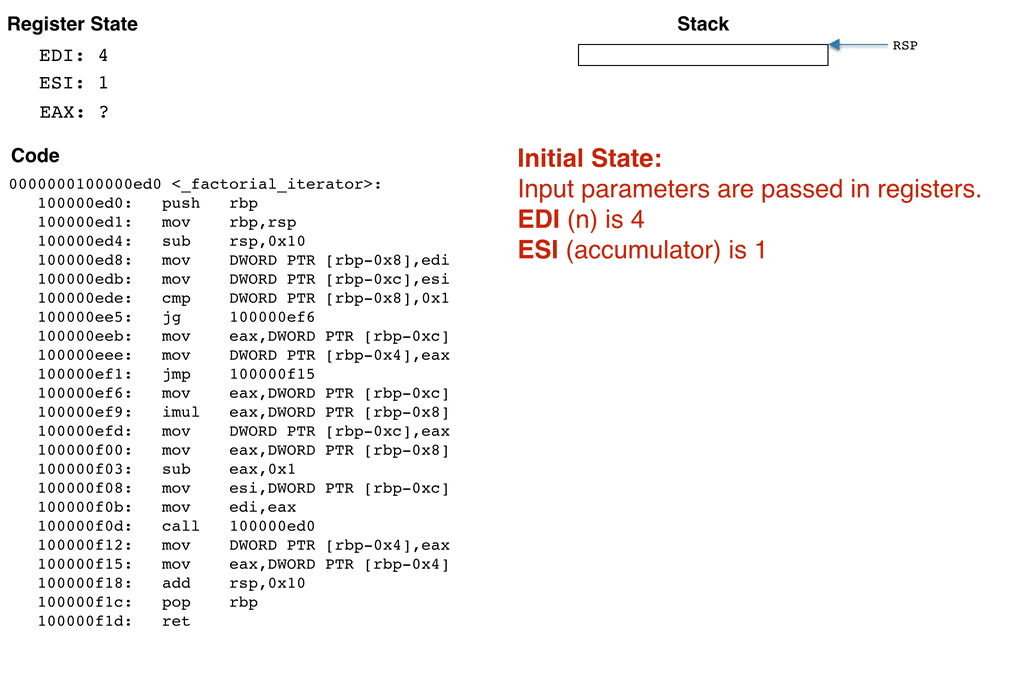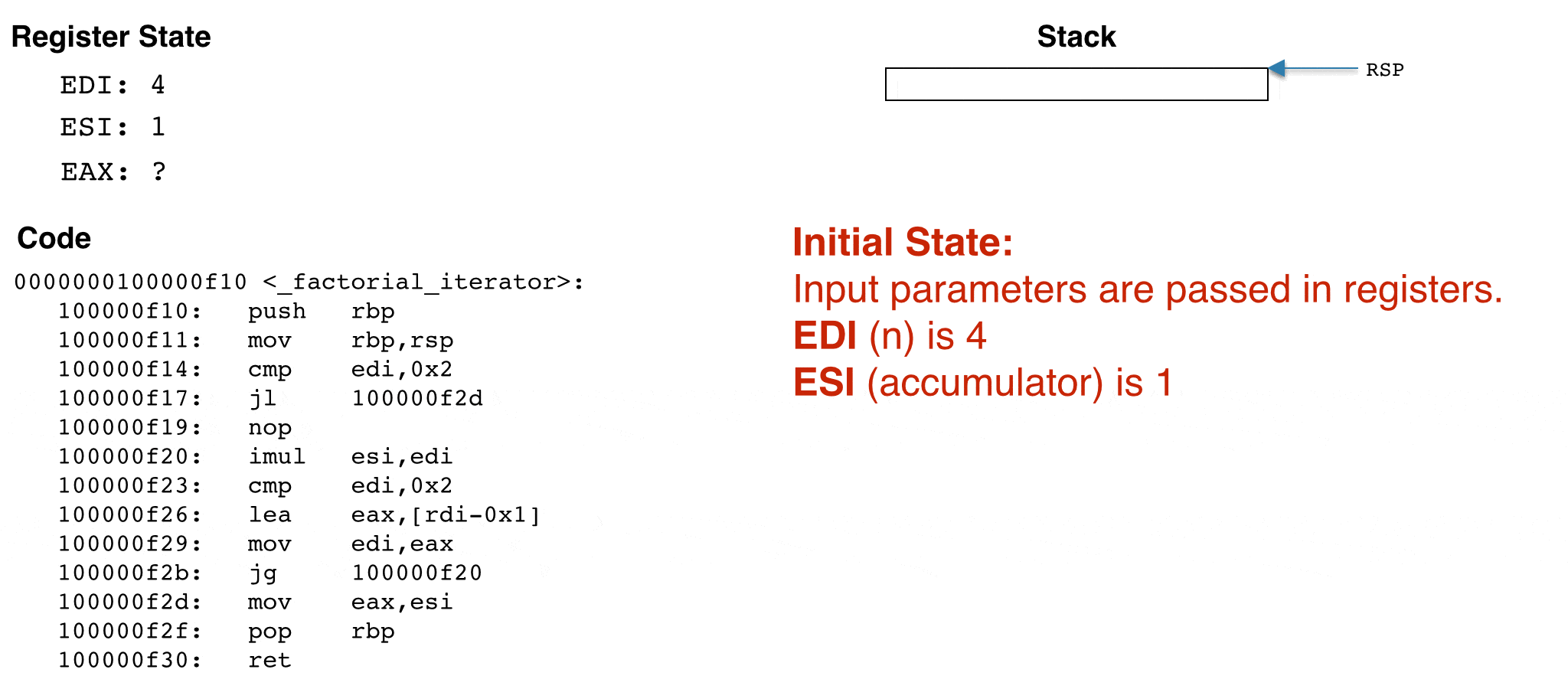# Optimizing Tail Call Recursion

### Article summary

I have been familiar with the concept of tail recursion for some time, though I had never explored it in depth. In this post I’ll look at an example of what happens when a tail recursive function is optimized on x86 targeting OS X.

## Recursion Limitations

Recursion is common in software, especially in functional programming languages. Unlike iteration (e.g., while or for loops), recursion has the unfortunate side effect of growing the execution stack (a finite region of memory that a program uses during its execution). This can cause problems when many repeated recursive calls are made, as the stack may run out of space. It’s also slower than iteration due to the extra stack frame creation and tear down.

Luckily, certain types of recursive calls can be re-written to execute in an iterative manner where they use constant stack space. Whether or not this optimization happens depends on the structure of the recursive call, the language, the compiler, and compiler options used.

## Understanding Tail Calls

Let’s start by talking about tail calls. According to Wikipedia, a tail call is a function call that is made as the final action of a procedure. If the function is a recursive call, it is said to be tail recursive. Let’s look at a few examples.

### Example 1

```// Example 1
int foo(int x) {
return bar(y + 1);  // this is a tail call
}```

### Example 2

```int foo_recursive(int x) {
if (x > 0)
return foo_recursive(x - 1);  // this is a tail recursive call
}```

### Example 3

```int foo_not_tail_call(int x){
if (x > 0)
return 1 + foo_not_tail_call(x - 1);  // this is not a tail call
}
```

In Example 1, the function call to `bar` is a tail call. In Example 2, `foo_recursive` is a recursive tail call, making it an example of tail recursion. In Example 3, `foo_not_tail_call` is not a tail call because there is an addition operation (+ 1) that happens after the call returns. I’ve found that some compilers can optimize the third scenario, probably by re-writing it to look more like Example 2, though for the sake of this post let’s ignore the Example 3 scenario. General tail call optimization is a complex subject. To keep things simple we’ll focus on tail recursion in this post (e.g., Example 2 from above).

## Tail Recursion Deep Dive

With that general idea in mind, let’s look at an example and see how it gets optimized. Below is a `factorial` function that calls a recursive `factorial_iterator` function. The `factorial_iterator` function calls itself with a decreasing n value and an accumulator. This accumulator model is a common technique used to make a function tail recursive (and eligible for optimization).

```int factorial(int n) {
return factorial_iterator(n, 1);
}

int factorial_iterator(int n, int accumulator) {
if(n <= 1){
return accumulator;
} else {
accumulator = accumulator * n;
return factorial_iterator(n - 1, accumulator);
}
}
```

I compiled this example using clang/LLVM on OS X Mavericks using no compiler optimizations, and have included a disassembly of the code below. I've annotated what each line is doing with some comments. For a more detailed description of each x86 instruction, refer to Volume 2 of the Intel Software Developers Manual. It's important to note that depending on the compiler you're using, the platform you're targeting, its calling conventions, etc., your disassembly might look quite a bit different than mine.

```0000000100000f20 <_factorial>:                // Input: edi contains n
100000f20:   push   rbp                      // save stack base ptr
100000f21:   mov    rbp,rsp                  // setup frame base ptr
100000f24:   sub    rsp,0x10                 // move stack ptr to end of frame
100000f28:   mov    esi,0x1                  // store 1 in esi
100000f2d:   mov    DWORD PTR [rbp-0x4],edi  // move edi (accumulator variable) to the stack
100000f30:   mov    edi,DWORD PTR [rbp-0x4]
100000f33:   call   100000ed0                // save instruction ptr, jmp to factorial_iterator
100000f38:   add    rsp,0x10                 // collapse stack frame
100000f3c:   pop    rbp                      // restore stack base ptr
100000f3d:   ret                             // return, result is in eax

0000000100000ed0 <_factorial_iterator>:       // Input: edi (n), esi (accumulator)
100000ed0:   push   rbp                      // save previous stack base pointer
100000ed1:   mov    rbp,rsp                  // setup frame base ptr
100000ed4:   sub    rsp,0x10                 // adjust sack ptr to end of stack frame
100000ed8:   mov    DWORD PTR [rbp-0x8],edi  // move edi (holds input param n) to the stack
100000edb:   mov    DWORD PTR [rbp-0xc],esi  // move esi (holds input param accumulator) to stack
100000ede:   cmp    DWORD PTR [rbp-0x8],0x1  // compare input param n with 1 (to set flags)
100000eeb:   mov    eax,DWORD PTR [rbp-0xc]  // else move accumulator to eax
100000eee:   mov    DWORD PTR [rbp-0x4],eax
100000ef1:   jmp    100000f15                // jmp to tear down
100000ef6:   mov    eax,DWORD PTR [rbp-0xc]  // move accumulator into eax
100000ef9:   imul   eax,DWORD PTR [rbp-0x8]  // accumulator = accumulator * n, result in eax
100000efd:   mov    DWORD PTR [rbp-0xc],eax  // put result on stack
100000f00:   mov    eax,DWORD PTR [rbp-0x8]
100000f03:   sub    eax,0x1                  // n = n -1
100000f08:   mov    esi,DWORD PTR [rbp-0xc]  // move current accumulator value into esi
100000f0b:   mov    edi,eax                  // move current n value into edi
100000f0d:   call   100000ed0                // save instruction ptr on stack and jmp
100000f12:   mov    DWORD PTR [rbp-0x4],eax  // return from recursive call
100000f15:   mov    eax,DWORD PTR [rbp-0x4]  // move the result to eax
100000f18:   add    rsp,0x10                 // collapse stack frame
100000f1c:   pop    rbp                      // restore stack base ptr
100000f1d:   ret                             // return, result is in eax
```

There are a few nuances that are important to understand in the above disassembly. First, all input parameters and return values are passed in registers (not on the stack). The input parameter `n` is passed into `_factorial` via the `edi` register. Similarly, `n` is also passed into `_factorial_iterator` via `edi`, and `accumulator` is passed in via `esi`. All returns values are returned in the `eax` register.

The `_factorial_iterator` function makes recursive calls to itself. This can be observed via the `call 1000000ed0` line. Each call creates a new stack frame (also called activation record) for the newly-called function to use for its execution.

### Non-optimized example

To help visualize how the stack grows and what happens to the stack and register state when the above code is run, I've created an animation that computes 4 factorial (e.g., n = 4). The animation steps through `_factorial_iterator` and displays the stack and register state after each instruction. Recall that the input n, which is 4 for this example, is passed in via the `edi` register.I tried to make the above animation as accurate as possible while still capturing the fundamentals of how the register state and stack changes as the code executes. The most important take away is that the stack grows for each recursive call. The animation computes 4!, and results in four stack frames being produced. You can imagine how larger inputs would result in the stack consuming a lot of memory. For sufficiently large inputs, the stack can easily run out of space, resulting in a stack overflow.

### Optimized example

Now that we've seen what happens under normal (non-optimized) operating conditions, let's look at an optimized example. Enabling tail call optimization for clang can be achieved via the `-O1` optimization level flag. The process is similar for gcc. The optimized disassembly looks like this.

```0000000100000f40 <_factorial>:          // Input: edi contains n
100000f40:   push   rbp                // save stack base ptr
100000f41:   mov    rbp,rsp            // setup frame base ptr
100000f44:   mov    esi,0x1            // move 1 to esi (initialize accumulator)
100000f49:   pop    rbp                // restore stack base ptr
100000f4a:   jmp    100000f10          // jmp directly to factorial_iterator

0000000100000f10 <_factorial_iterator>: // Input: edi contains n, esi contains accumulator
100000f10:   push   rbp                // save stack base ptr
100000f11:   mov    rbp,rsp            // setup frame base ptr
100000f14:   cmp    edi,0x2            // compare n to 2
100000f17:   jl     100000f2d          // if n < 2 jmp to 0x10000f2d
100000f19:   nop
100000f20:   imul   esi,edi            // accumulator = accumulator * n
100000f23:   cmp    edi,0x2            // compare n and 2
100000f26:   lea    eax,[rdi-0x1]      // eax = n - 1
100000f29:   mov    edi,eax            // n = eax
100000f2b:   jg     100000f20          // if n > 2 jmp to 0x10000f20 (flags still set)
100000f2d:   mov    eax,esi            // move n to eax
100000f2f:   pop    rbp                // restore base ptr
100000f30:   ret                       // return, result is in eax
```

This time around the assembly code looks a lot different. Notice that we don't even call `factorial_iterator` from factorial. Instead, we just `jmp` directly to it. Similarly, there are no `call` instructions in `factorial_iterator`. In fact, there is no stack writing/reading at all!. Instead, all computations are performed via register operations that amount to something that looks like a while loop. For completeness, here is the much simplified optimized animation.As we would expect, there is no stack activity. The total number of instructions between the original code example and the optimized example is almost reduced by a factor of four.

## Details

For those interested, all the examples in this post were compiled with clang/LLVM on OSX Mavericks. The LLVM version is:

```Apple LLVM version 6.0 (clang-600.0.51) (based on LLVM 3.5svn)
Target: x86_64-apple-darwin14.0.0
```

I used gobjdump to disassemble the compiled binaries and display them using Intel assembly syntax.

### Related Posts

• Software Science

## ChatGPT and the Value of a Computer Science Education

• Software Science

## Inspired by Nature: An Introduction to Genetic Algorithms

• Software Science

## Learn the Fascinating History and Uses of the Public Suffix List

Conversation
•Xin Huang says:

Interesting article. Like those animations. Wondering how did you make it?